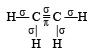Courses

# 28 Year NEET Questions: Chemical Bonding And Molecular Structure- 1

## 39 Questions MCQ Test Chemistry 28 Years Past year papers for NEET/AIPMT Class 11 | 28 Year NEET Questions: Chemical Bonding And Molecular Structure- 1

Description
This mock test of 28 Year NEET Questions: Chemical Bonding And Molecular Structure- 1 for NEET helps you for every NEET entrance exam. This contains 39 Multiple Choice Questions for NEET 28 Year NEET Questions: Chemical Bonding And Molecular Structure- 1 (mcq) to study with solutions a complete question bank. The solved questions answers in this 28 Year NEET Questions: Chemical Bonding And Molecular Structure- 1 quiz give you a good mix of easy questions and tough questions. NEET students definitely take this 28 Year NEET Questions: Chemical Bonding And Molecular Structure- 1 exercise for a better result in the exam. You can find other 28 Year NEET Questions: Chemical Bonding And Molecular Structure- 1 extra questions, long questions & short questions for NEET on EduRev as well by searching above.
QUESTION: 1

### In an octahedral structure, the pair of d orbitals involved in d2sp3 hybridization is 

Solution:

Only those d or bital s whose  l obes are directed along X, Y and Z directions hybridise with s and p orbitals. In other three d or bitals n amely dxy, dyz and dxz, the lobes are at an angle of 45° from both axis, hence the extent of their overlap with s and p orbitals is much lesser than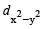and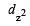orbitals.

QUESTION: 2

### In BrF3 molecule, the lone pairs occupy equatorial positions to minimize 

Solution: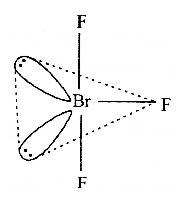Bent T-shaped geometry in which both lone pairs occupy the equatorial positions of the trigonal bipyramid. Here (lp - lp) repulsion = 0, (lp - bp) repulsion = 4 and (bp - bp) repulsion = 2.

QUESTION: 3

### Which of the following is the electron deficient molecule? 

Solution:

Molecules containing less than two valence electrons per bond are referred to as electron deficient.

B2H6B2H6 molecule has total 8 covalent bonds but only 12 electrons i.e. 6 pairs. Hence it has 2 electron pairs less than maximum required number for bonding, which makes it electron deficient.

B2H6B2H6 is called diborane. In this compound, the octet for B is incomplete which is why it dimerizes. So B2H6B2H6 is electron deficient.

QUESTION: 4

The correct sequence of increasing covalent character is represented by 

Solution:

As difference of electronegativity increases % ionic character increases and covalent character decreases i.e., negativity difference decreases covalent character increases.
Further greater the charge on the cation more will be its covalent character. Be has maximum (+2) charge.

QUESTION: 5

Which of the following molecules has trigonal planar geometr y? 

Solution:

3 is sp2 hybridised. So, it is trigon al planner. NH3, PCl3 has sp3 hybridisation hence has trigonal bipyramidal shape, IF3, has sp3d hydridization and has linear shape.

QUESTION: 6

Which of the following would have a permanent dipolemoment? 

Solution:

SF4 has permanent dipole moment.
SF4 has sp3d hybridization and see saw shape (irregular geometry).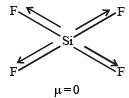Whereas XeF4 shows squre planar geometry SiF4 has tetrahedral shape and BF3 has Trigonal planar shape. All these are symmetric molecules. Hence μ ≠ 0.

QUESTION: 7

Which of the following is not a correct statement?

Solution:

Statement (a), (c), (d) are correct. Statement (b) is incorrect statement.
AB5 may have two structures as follows :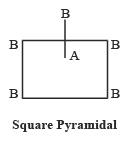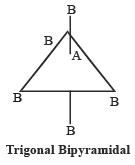QUESTION: 8

The number of unpaired electrons in a paramagnetic diatomic molecule of an element with atomic number 16 is 

Solution:

Electronic configuration of the molecule according to molecular orbital theory, is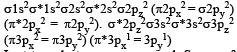Last two electrons are unpaired. So no. of unpaired electron is 2.

QUESTION: 9

In which of the following molecules all the bonds are not equal? 

Solution:

In BF3, AlF3 & NF3 all fluoride atoms are symmetrically oriented with respect to central metal atom but in ClF3 three fluorine atoms are arranged as follows :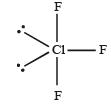Here two bonds are in equitorial plane & one bond is in axial plane.

QUESTION: 10

The electronegativity difference between N and F is greater than that between N and H yet the dipole moment of NH3 (1.5 D) is larger than that of NF3 (0.2D). This is because 

Solution:

In NH3 the atomic dipole and bond dipole are in the same direction whereas in NF3 these are in opposite direction so in the former case they are added up whereas in the latter case net result is reduction of dipole moment. It has been shown in the following figure :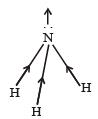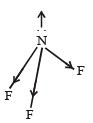QUESTION: 11

Which of the following species has a linear shape ?

Solution:

NO2+ will have linear shape as it will have sp hybridisation.

QUESTION: 12

Which of the following is not isostructural with SiCl4?

Solution:

In SiCl4 there is sp3 hybridisation so the structure is tetrahedral. In SO42–, PO43–, NH4+ the structure is tetrahedral because in all hybridisation is sp3. But in SCl4, sp3d hybridisation present so shape is different which is see saw.

QUESTION: 13

In which of the following pairs, the two species are iso-structure?

Solution:

Hybridization of Br is BrO3
Total valence elctrons = 7 + 3 × 6 = 25
Charge = –1 hence
Total = 25 + 1 = 26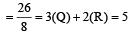hybridization = dsp3 hybridization of Xe in XeO3 Total valence electrons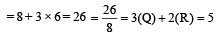Hybridization = dsp3 In both cases, the structure is trigonal pyramidal.

QUESTION: 14

The correct order of C–O bond length among CO, CO32- , CO2 is 

Solution:

All these structures exhibits resonance and can be represented by the following resonating structures.

(i)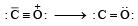(ii)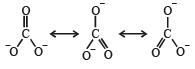(iii)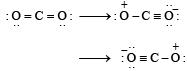More is the single bond character. More will be the bond length. Hence, the corret order is :

CO < CO2 < CO3– –

QUESTION: 15

The angular shape of ozone molecule (O3) consists of : 

Solution:

Single bond contains = 1 σ bond
Double bond contains = 1 σ bond + 1 π bond
Triple bond contains = 1σ  bond + 2 π bond

The molecule of O3 is bent with an angle of 116.8o and equal O-O distance of 128 pm. The ozone molecule consists of 2 sigma bond and 1 pi bond.

QUESTION: 16

The correct order of increasing bond angles in the following triatomic species is : 

Solution:

From the structure of three species we can determine the number of lone pair electrons on central atom (i.e. N atom) and thus the bond angle.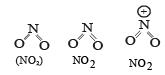We know that higher the number of lone pair of electron on central atom, greater is the lp – lp repulsion between Nitrogen and oxygen atoms. Thus smaller is bond angle..
The correct order of bond angle is

NO2- <NO2<NO2 i .e. opti on (b) is correct.

QUESTION: 17

Four diatomic species are listed below in different sequences. Which of these presents the correct order of their increasing bond order ? 

Solution:

Calculating the bond order of various species.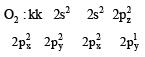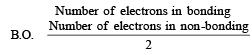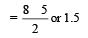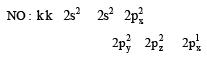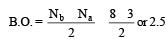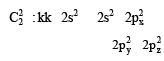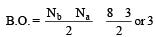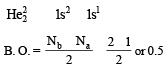From these values we find the correct order of increasing bond order is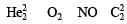QUESTION: 18

What is the dominant intermolecular force or bond that must be overcome in converting liquid CH3OH to a gas? 

Solution:

Due to intermolecular hydrogen bonding in methanol, it exist as assosiated molecule.

QUESTION: 19

In which of the following molecules / ions BF3, NO2-, NH2- and H2O ,  the central atom is sp2 hybridized ?

Solution:

For neutral molecules: No. of electron pairs = No. of atoms bonded
to it +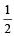[Group number of central atom –Valency of the central atom].
For ions : No. of electron pairs = No. of atoms bonded
to it +[Group no. of central atom – valency of the central atom ± no. of electrons] On calcuting no. of electron pairs in given molecules. We find that in the given molecules hybridisation is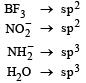QUESTION: 20

In which of the following pairs of molecules/ ions, the central atoms have sp2 hybridization? 

Solution: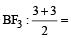3 means sp2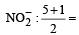3 means sp2

QUESTION: 21

In which one of the following species the central atom has the type of hybridization which is not the same as that present in the other three?

Solution: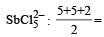6 means sp3d2

I3 , SF4 , and PCl5 ; all have sp3d hybridization.

QUESTION: 22

Some of the properties of the two species, NO3- and H3O+ are described below. Which one of them is correct? 

Solution:

In NO3-2 , nitrogen is in sp2 hybridisation, thus planar in shape. In H3O+ , oxygen is in sp3 hybridisation, thus tetrahedral in shape.
∴ Correct choice : (b)

QUESTION: 23

In which of the following molecules the central atom does not have sp3 hybridization? 

Solution:

(a) NH+4  : sp3 hybridisation
(b) CH4: sp3 hybridisation
(c) SF4: sp3 d hybridisation
(d) BF4 : sp3 hybridisation
∴ Correct choice : (c)

QUESTION: 24

Considering the state of hybridization of carbon atoms, find out the molecule among the following which is linear ? 

Solution: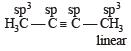QUESTION: 25

Which of the two ions from the list given below that have the geometry that is explained by the same hybridization of orbitals, NO2, NO3, NH2, NH4+, SCN ? 

Solution:

Hybridisation =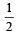[No. of valenceelectrons of central atom + no. of monovalent atoms attached to it – Negative charge if any – positive charge if any]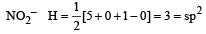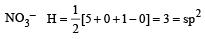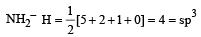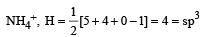SCN  = sp i.e., NO2and NO3 have same hybridisation.

QUESTION: 26

Which one of the following pairs is isostructural (i.e., having the same shape and hybridization)? 

Solution:

BF4- hybridisation sp3, tetrahedral  structure.
NH4+ hybridisation sp3, tetrahedral structure.

QUESTION: 27

Bond order of 1.5 is shown by : 

Solution:

(O2) =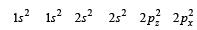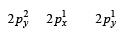Bond order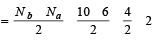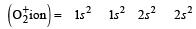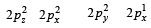Bond order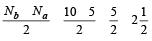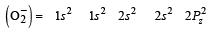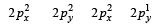Bond order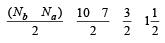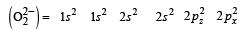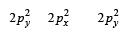Bond order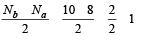QUESTION: 28

Which of the following species contains three bond pairs and one lone pair around the central atom ?

Solution: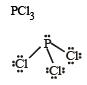QUESTION: 29

The pair of species with the same bond order is : 

Solution:

Both O22– and B2 has bond order equal to 1.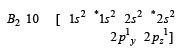Bondorder =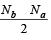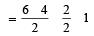B2 is known in the gas phase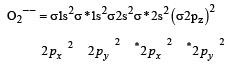Bond order  =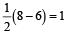QUESTION: 30

During change of O2 to O2- ion, the electron adds on which one of the following orbitals ? [2012 M]

Solution: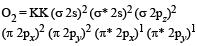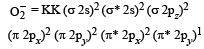QUESTION: 31

Four diatomic species are listed below. Identify the correct order in which the bond order is increasing in them: [2012 M]

Solution:

Bond order  =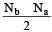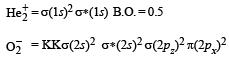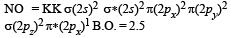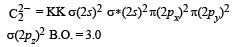QUESTION: 32

Which of the following is electron - deficient ? [NEET 2013]

Solution: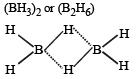It contains two 3 centre - 2 electron bonds and present above and below the plane of molecules compounds which do not have sufficient number of electrons to form normal covalent bonds are called electron deficient molecules.

QUESTION: 33

Which one of the following molecules contains no pi -bond?

[NEET 2013]

Solution:

All the molecules have O atom with lone pairs, but in H2O the H atom has no vacant orbital for pi-bonding. That's why it does not have any pi- bond.

In all other given molecules, the central atom because of the presence of vacant orbitals is capable to farm Pi- bonds.

QUESTION: 34

Which of the following is paramagnetic ? [NEET 2013]

Solution:

Molecular orbital configuration of O2- is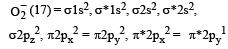QUESTION: 35

XeF2 is isostructural with : [NEET 2013]

Solution: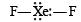sp3d and Linear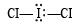sp3d and Linear

QUESTION: 36

In which of the following pair both the species have sp3 hybridization? [NEET Kar. 2013]

Solution:

Applying VSEPR theory, both NF3 and H2O are sp3 hybridized.

QUESTION: 37

The pair of species that has the same bond order in the following is: [NEET Kar. 2013]

Solution:

No. of electrons in CO = 6 + 8 = 14 No. of electrons in NO+ = 7 + 8 – 1 = 14
∴ CO and NO+ are isoelectronic species.
Isoelectronic species have identical bond order.

QUESTION: 38

In which of the following ionisation processes the bond energy increases and the magnetic behaviour changes from paramagnetic to diamagnetic? [NEET Kar. 2013]

Solution:

A) Going from N2 to N2+, bond order decreases from 3 to 2.5
B) Going from O2 to O2+, bond order increases from 2 to 2.5, but magnetic behavior does not change. Both of these species are paramagnetic.
C) Going from C2 to C2+, bond order decreases from 2 to 1.5.
D) Going from NO to NO+, bond order increases from 2.5 to 3, and the magnetic behavior changes from paramagnetic to diamagnetic.
So, option d is correct.

QUESTION: 39

The outer orbitals of C in ethene molecule can be considered to be hybridized to give three equivalent sp2 orbitals. The total number of sigma (σ) and pi (π) bonds in ethene molecule is [NEET Kar. 2013]

Solution: